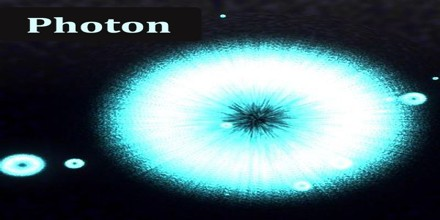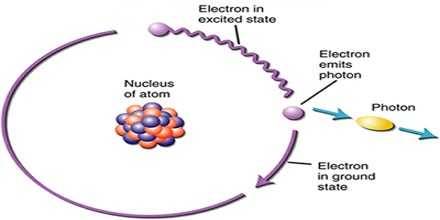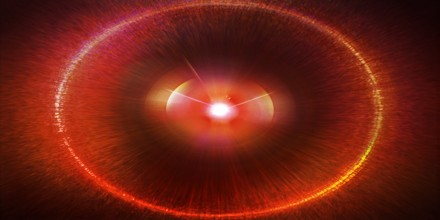Definition

A photon (also called light quantum) is the quantum of electromagnetic radiation. The term quantum is the smallest elemental unit of a quantity, or the smallest discrete amount of something. Thus, one quantum of electromagnetic energy is called a photon. The plural of quantum is quanta. The concept originated (1905) in Albert Einstein’s explanation of the photoelectric effect, in which he proposed the existence of discrete energy packets during the transmission of light.

The term photon (meaning “visible-light particle”) was coined for energy packets. Particle-like behavior is not restricted to the visible-light portion of the electromagnetic radiation spectrum, however. Radio waves, infrared rays, visible light, ultraviolet rays, X rays, and gamma rays all consist of photons, each of which contains a particular amount of energy that depends on the wavelength.The concept of photons and quanta comes from quantum mechanics and quantum theory. Quantum mechanics is a mathematical model that describes the behavior of particles on an atomic and subatomic scale. It demonstrates that matter and energy are quantized, or come in small discrete bundles, on the smallest scales imaginable. A photon propagates at the speed of light.

Photons travel through empty space at a speed of approximately 186,282miles (299,792 kilometers) per second. This is true no matter what the electromagnetic wavelength. In media other than a vacuum, the speed is reduced. For example, visible light travels more slowly through glass than through outer space. Radio waves travel more slowly through the polyethylene in a transmission line than they do through the atmosphere. The ratio of the speed of the photons in a particular medium to their speed in a vacuum is called the velocity factor. This factor is always between 0 and 1 (or 0 and 100 percent), and it depends to some extent on the wavelength.

Energy of Photons

The idea of quantum mechanics and photons originated from scientists’ observations of the photoelectric effect. The photoelectric effect is where light striking a metal surface causes electrons to be ejected from the metal. Scientists were unable to explain this phenomenon, but eventually the explanation came from quantum theory.What they found was that the energy in each quantum of light depends on the frequency of the light. In particular, the energy of a photon equals Planck’s constant times the frequency of the radiation. Mathematically, this is given by the equation E = hf. Planck’s constant is the fundamental constant of quantum theory that determines the scale of the small-scale world. Planck’s constant = 6.63 * 10-34 joule-second (J-s). The total energy in an electromagnetic wave is the sum of the energies of each photon in the wave.

The energy of a photon is so small that we usually measure it in electronvolts (eV). One eV is the potential energy of each electron in a 1-volt battery. One eV is equal to 1.6 * 10-19 joules (J). Therefore, we need to convert Planck’s constant to appropriate units, which are electronvolts/hertz (eV/Hz). In eV/Hz, Planck’s constant is 4.136 * 1015 eV/Hz.

The energy contained in a single photon does not depend on the intensity of the radiation. At any specific wavelength — say, the wavelength of light emitted by a helium-neon laser — every photon contains exactly the same amount of energy, whether the source appears as dim as a candle or as bright as the sun. The brilliance or intensity is a function of the number of photons striking a given surface area per unit time.Applications of Photon

Individual photons can be detected by several methods. The classic photomultiplier tube exploits the photoelectric effect: a photon of sufficient energy strikes a metal plate and knocks free an electron, initiating an ever-amplifying avalanche of electrons. Semiconductor charge-coupled device chips use a similar effect: an incident photon generates a charge on a microscopic capacitor that can be detected. Other detectors such as Geiger counters use the ability of photons to ionize gas molecules contained in the device, causing a detectable change of conductivity of the gas.Photons have many applications in technology. These examples are chosen to illustrate applications of photons per se, rather than general optical devices such as lenses, etc. that could operate under a classical theory of light. The laser is an extremely important application and is discussed above under stimulated emission.

Several different kinds of hardware random number generators involve the detection of single photons. In one example, for each bit in the random sequence that is to be produced, a photon is sent to a beam-splitter. In such a situation, there are two possible outcomes of equal probability. The actual outcome is used to determine whether the next bit in the sequence is “0” or “1”.